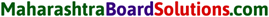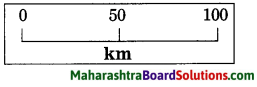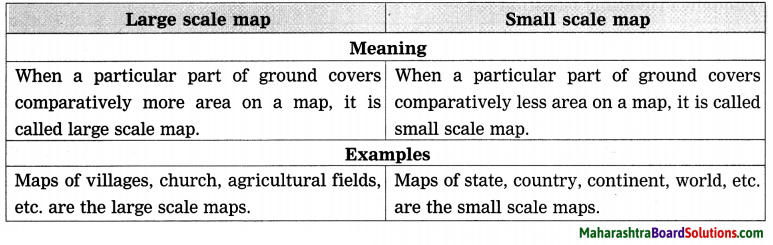# Maharashtra Board Class 8 Geography Solutions Chapter 9 Map Scale

Balbharti Maharashtra State Board Class 8 Geography Solutions Chapter 9 Map Scale Notes, Textbook Exercise Important Questions and Answers.

## Maharashtra State Board Class 8 Geography Solutions Chapter 9 Map Scale

Class 8 Geography Chapter 9 Map Scale Textbook Questions and Answers

1.

Map Scale Std 8 Question a.
Classify maps showing the following areas into small scale or large scale:
(1) Building (2) School (3) Country of India (4) Church (5) Mall (6) World map (7) Garden (8) Dispensary (9) Maharashtra state (10) The north sky at night.
The classification of maps showing the given areas is as follows:
(A) Small scale maps:

1. Country of India
2. World map
3. Maharashtra state
4. The north sky at night.

(B) Large scale maps :

1. Building
2. School
3. Church
4. Mall
5. Garden!
6. Dispensary.Map Scale Class 8 Geography Question b.
There are two maps with respective scales of 1cm = 100 m and 1cm = 100 km. Give well reasoned answer as to which of them would be a large scale map and which a small scale map. Recognize the types of maps.
A. Out of the two maps with respective scales of 1 cm = 100 m and 1 cm = 100 km, a map with respective scale of 1 cm = 100 m would be a large scale map.
B. Reasons :

1. 1 metre is equal to 100 centimetres and 100 metre is equal to 10000 centimetres.
2. Thus, the value of the given verbal scale (1cm = 100 m) is 1 : 10000 in numerical terms (scale).
3. A map having a numerical scale of 1 : 10,000 or less than it is called large scale map. Therefore, 1cm = 100 m would be a large scale map.

C. Types of maps :

1. Maps of villages, church, agricultural fields, etc. are the large scale maps.
2. Maps of state, country, continent, world, etc. are the small scale maps.

2. Using a map of India from the atlas measure straight Line distance between the following cities and complete the table below.

Question a.Cities Distance on a map Actual distance 1. Mumbai to Bangaluru 0.98 cm 980 km 2. Vijaypura to Jaipur 2 cm 2000 km 3. Hyderabad to Surat 0.9 cm 900 km 4. Ujjain to Shimla 1.14 cm 1140 km 5. Patna to Raipur 0.75 cm 750 km 6. Delhi to Kolkata 1 cm 1000 km3.

Map Scale Std 8 Questions And Answers Question a.
The distance between two points A and B on the ground is 500 m. Show this distance on paper by a line of 2 cm. Express the map scale by any one method and mention it.Question b.
Convert verbal scale of 1cm = 53 km to a numerical scale.

1. 1 kilometre is equal to 100000 centimetres. Therefore, 53 kilometres is equal to 5300000 centimetres.
2. Therefore, the verbal scale of 1 cm = 53 km can be converted to a numerical scale as – 1 : 5300000.Class 8 Geography Chapter 9 Map Scale Question c.
Convert numerical scale of 1 : 10000000 to a verbal scale in the metric system.

1. 100000 centimetres is equal to 1 kilometre. Thus, 10000000 centimetres is equal to 100 kilometres.
2. Therefore, numerical scale of 1 : 10000000 to a verbal scale in the metric system can be converted as 1 cm = 100 km.

4. Help them, using road and railway maps of the state of Maharashtra. Use the scale given in the maps.

Std 8 Geography Chapter 9 Map Scale Question a.
Ajay wants to arrange a family trip. Beed-Aurangabad-Dhule-Nasik Mumbal-Pune-Solapur-Beed. He wants to visit tourist places along this route. The cost of the vehicle is Rs 12/- per km. What would be the approximate cost of travel?

Map Scale Questions And Answers Question b.
Saloni has been asked to organize a trip by her teacher. She has selected Nagpur Chandrapur-Nanded-Washim-Akola Malkapur. What would be the total coverage in kilometers?

Geography Class 8 Chapter 9 Question c.
Vishawasrao is transporting goods in a vehicle from Alibag (district Raigad) to Naldurg (district – Osmanabad). How many km. will he be covering aproximately for a to and fro travel?

Projects:

Map Scale Questions Question a.
Measure the length and breadth of your school. Prepare a sketch according to scale. Show different parts of your school on the sketch.

Maharashtra State Board Class 8 Geography Solutions Question b.
With the help of google maps find the distance between your village and your neighbouring village. Represent all the three methods of map scale on paper.

Class 8 Geography Chapter 9 Map Scale Additional Important Questions and Answers

Mark ✓ the box next to the right alternative:
(Note: The answers are given directly.)

Question a.
Which of the following factor’s map will be a large scale map?
(a) Temple [ ]
(b) State [ ]
(c) Nation [ ]
(d) Continent [ ]
(a) Temple [✓]Question b.
Which of the following scale indicates small scale map?
(a) 1 : 100 [ ]
(b) 1 : 1000 [ ]
(c) 1 : 10000 [ ]
(d) 1 : 100000 [ ]
(d) 1 : 100000 [✓]

Answer the following questions in one sentence each:

Question a.
What is verbal scale?
A scale in which distances are expressed with the use of words indicating measurement is called verbal scale.

Question b.
What is numerical scale?
A scale in which distances are expressed as ratio is called numerical scale.

Question c.
What is linear scale?
A scale in which distances are expressed by drawing graphical scale is called linear scale.Question d.
What is large scale map?
A map in which a particular part of ground covers comparatively more area is called large scale map.

Question e.
What is small scale map?
A map in which a particular part of ground covers comparatively less area is called small scale map.

Write short notes on:

Question a.
Verbal scale.
1. A scale in which distances are expressed with the use of words indicating measurement is called verbal scale.
2. For example, 1cm = 100 km.

3. In verbal scale, the word indicating measurement on the left hand side indicates the distance between any two points on a s map. On the other hand, the word indicating 1 measurement on the right hand side! indicates the ground distance between those two points.

4. When the map is reduced or enlarged by taking its photo copy, the verbal scale on the original map does not change.

Question b.
Numerical scale.

1. Numerical scale: A scale in which distances are expressed as ratio is called numerical scale.
2. For example, 1:10000. It is also known as representative fraction.
3. In numerical scale, the same measuring unit is used for the figures on the left hand side and right hand side. However, no words are used to indicate this measuring unit.
4. In numerical scale, number 1 on the left hand side indicates the distance between any two points on a map. On the other hand, the number 10000 on the right hand side indicates the ground distance between those two points.
5. When the map is reduced or enlarged by taking its photo copy, the numerical scale on the original map does not change.Question c.
Linear scale.

1. A scale in which distances are expressed by drawing graphical scale is called linear scale.
2. For example,3. Compass or blade of grass is used if the ruler is not available for the measurement.
4. A thread is used for measuring the curved distances between two points shown in a map.
5. When the map is reduced or enlarged by taking its photo copy, the linear scale drawn on the original map changes as per the changing size of the map.

Highlight differences /Distinguish between the following:

Question a.
Large scale map and Small scale map.Question b.
Numerical scale and Linear scale.Study the following map /figure/graph and answer the following questions:Study the Figure and answer the following questions:

Question a.
How much is the ground distance between Mumbai and Gondia?
The ground distance between Mumbai and Gondia is approximately 810 kilometres.Question b.
How much is the distance between Satara and Sangli on a map?
The distance between Satara and Sangli on a map is approximately 1.5 centimetres.

Thought-Provoking Question:

Question a.
What is the need to use map scale? Think about it and write a paragraph.

1. If the map scale is not mentioned in a map, it will become difficult to know the ground (actual) distance between any two points shown in a map.
2. Map scale is important element of a map. It facilitates map reading.
3. If the map scale is mentioned in a map, it will become very easy to understand the ground (actual) distance between any two points shown in a map.

Open-Ended Question:

Question a.
Which of the following scale will you prefer to use: (a) Verbal scale (b) Numerical scale (c) Linear scale?